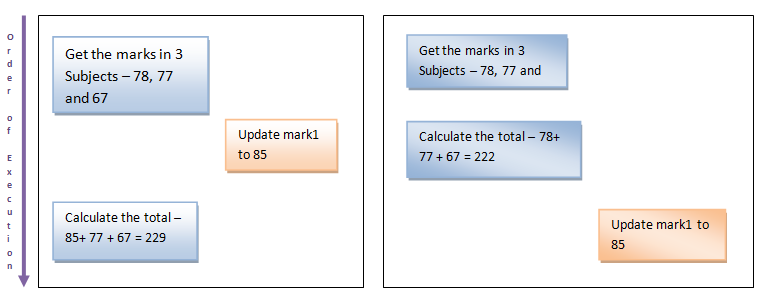# Schedules in DBMS

In a database system, we can have number of transaction processing. Related transactions will be processed one after another. There are some transactions processes in parallel. Some of the transactions can be grouped together.

Some of the transactions can be executed concurrently. That means, one transaction may be updating the data in the main memory while other transaction would be retrieving or updating the data in the secondary memory/ disk. This will increase the I/O of the transaction. Here no transaction has to wait for the other transaction to complete. Hence the performance / response time of the transaction is enhanced.

But this concurrent access will lead to wrong data read. For example, one transaction may be updating the data from the primary memory while other transaction will be trying to read the same data from the secondary memory which is not yet updated with new data. Hence the second transaction will have wrong value. Hence to make sure concurrent access to data does not create any inconsistency in the system, concurrent control mechanism is introduced. This mechanism makes sure that each transaction is executed in isolation and they do not induce any inconsistency in DB. It also defines a rule to execute transactions in a specific order, which is defined by a schedule.

A schedule is a process of grouping the transactions into one and executing them in a predefined order. A schedule is required in a database because when some transactions execute in parallel, they may affect the result of the transaction – means if one transaction is updating the values which the other transaction is accessing, then the order of these two transactions will change the result of second transaction. Hence a schedule is created to execute the transactions. A schedule is called serial schedule, if the transactions in the schedule are defined to execute one after the other.

Even when we are scheduling the transactions, we can have two transactions in parallel, if they are independent. But if they are dependent by any chance, then the results will change. For example say one transaction is updating the marks of a student in one subject; meanwhile another transaction is calculating the total marks of a same student. If the second transaction is executed after first transaction is complete, then both the transaction will be correct. But what if second transaction runs first? It will have wrong total mark.This type of incorrect processing of transaction needs to be handled in the system. Parallel execution of transaction is allowed in the database only if there is any equivalence relation among the transactions. There are three types of equivalence relation among the transactions – Result, view and Conflict Equivalence.

### Result Equivalence

If the two transactions generate same result after their execution then it is called as result equivalence. For example, one transaction is updating the marks of Student X and the other transaction is to insert a new student. Here both the transactions are totally independent and their order of execution does not matter.  Whichever order they are executed, the result is the same. Hence it is called result equivalence transactions.

### View Equivalence

Two schedules are said to be view equivalence, if the transaction in one schedule is same as the one in other. That means, both the transactions in two schedules perform same tasks. For example, say schedule1 has transaction to insert new students into STUDENT table and second schedule has the transaction to maintain the old student records in a new table called OLD_STUDENT. In both the schedules student details are inserted, but different tables (it does not matter if it is same table). Such schedules are called as view equivalence schedule.

### Conflict Equivalence

In this case both schedules will have different set of transactions, but they would be accessing same data and one of the schedules will be inserting/updating the records. In this equivalence, both the schedules will have conflicting set of transactions. Above example of updating the marks of one student by one transaction and calculating the total marks at the same time is a conflicting equivalence.

In all these cases if the transaction is serialized, then the issues can be resolved. Let us discuss more about this in subsequent articles.

Translate »